Homework Help Question & Answers

# For a hydrogen-like atom, classify the electron transitions according to whether they result in the absorption or e...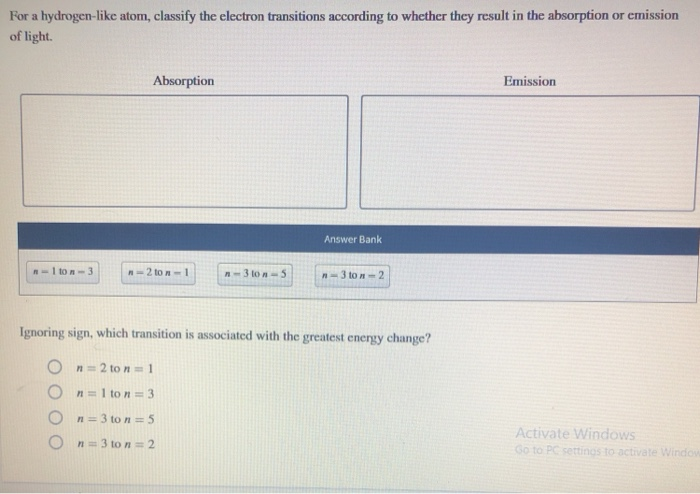For a hydrogen-like atom, classify the electron transitions according to whether they result in the absorption or emission of light. Absorption Emission Answer Bank -Iton-3 2 ton 3 - 5 - 3ton-2 Ignoring sign, which transition is associated with the greatest energy change? On=2 ton=1 o n =1 to n = 3 O n =3 to n = 5 O n =3 to n = 2 Activate Windows

#### Homework Answers

Answer #1 ✔ Recommended Answer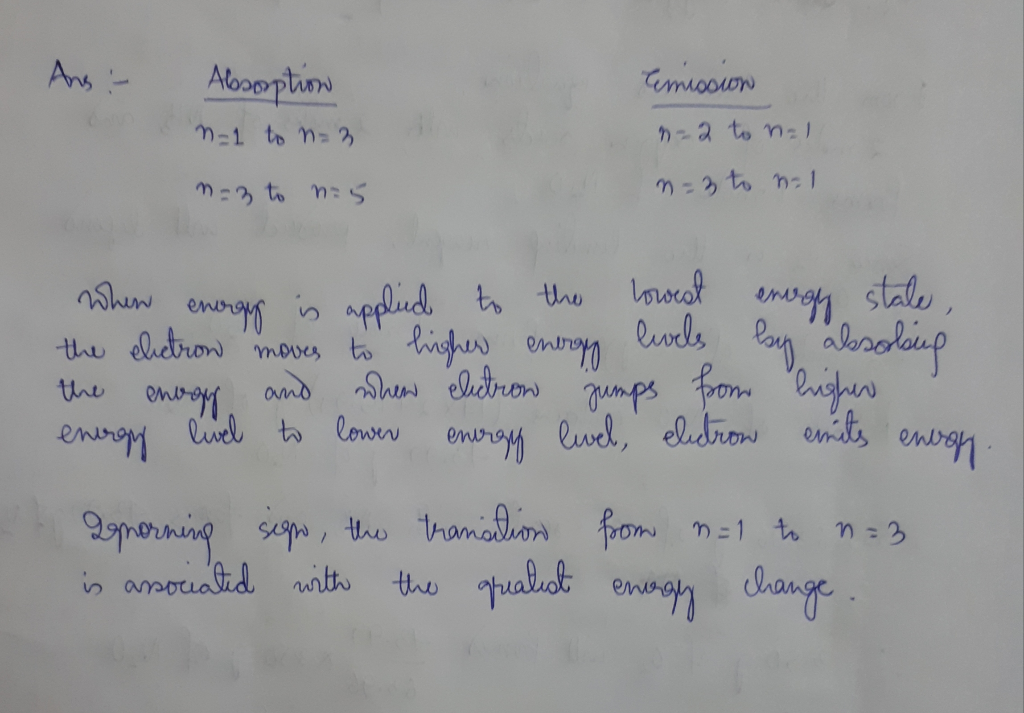Add a comment
Answer #2
Concepts and reason

An electronic transition takes place when electrons in a molecule undergo excitation or emission from one energy level to another energy level.

Fundamentals

When an electron is excited from lower energy level to the higher energy level some amount of energy is needed. The process is called as absorption.

When an electron is jumped from higher energy level to the lower energy level some amount of energy will be released. The process is called as emission.

The transition from n=1 to n=3 takes place from lower energy level to the higher energy level. Thus, this transition is absorption.

Electron transition takes place from n=2 to n=1. In the transition, n=1 is lower energy level and n=2 is a higher energy level.

The electronic transition is taking place from n=3 to n=2. The energy level n=2 is lower energy level and n=3 is a higher energy level.

In the transition from n=3 to n=5, n=3 is a lower energy level and n=5 is a higher energy level.

Identify the transition that takes place between the adjacent energy levels and between the far energy levels.

The transition from n=2 to n=1 takes place between the adjacent energy levels. The energy levels in the transition from n=3 to n=2 are also adjacent energy levels.

The energy levels in the transitions from n=1 to n=3 and from n=3 to n=5 are not adjacent energy levels. So these two transitions will have higher energy change. Of these two, n=1 to n=3 will have higher energy change.

Ans:

The transition from n=1 to n=3 is absorption.

The transition from n=2 to n=1 is emission.

The transition from n=3 to n=2 is emission.

The transition from n=3 to n=5 is absorption.

The transition from n=1 to n=3 is associated with greatest energy change.

Know the answer?
Your Answer:

#### Post as a guest

Your Name:

What's your source?

#### Earn Coin

Coins can be redeemed for fabulous gifts.

Not the answer you're looking for? Ask your own homework help question. Our experts will answer your question WITHIN MINUTES for Free.
Similar Homework Help Questions
• ### 2200 Resources Hint For a hydrogen-like atom, classify the electron transitions according to whether they result...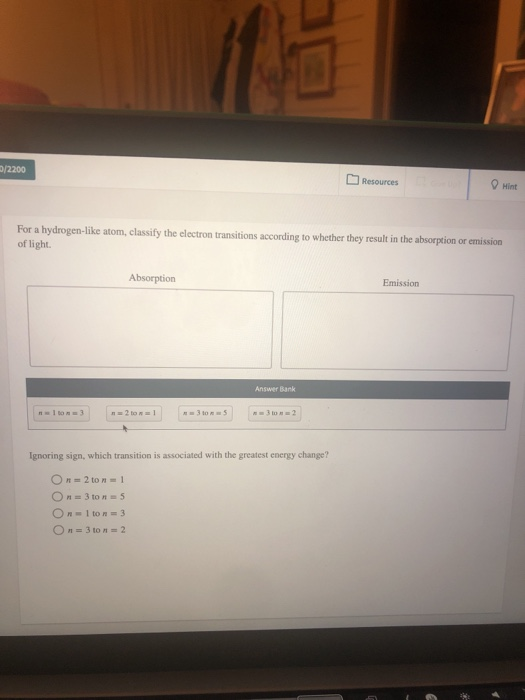2200 Resources Hint For a hydrogen-like atom, classify the electron transitions according to whether they result in the absorption or emission of light. Absorption Emission Answer Bank n1 to n3 3 to n n3 to n2 n2 to n 1 Ignoring sign, which transition is associated with the greatest energy change? On 2 to n1 On 3 to n 5 On-1 to n=3 On=3 to n2 Re re: 690/2200 22 How many photons are produced in a laser pulse of...

• ### A certain shade of blue has a frequency of 7.06 x 104 Hz. What is the energy of exactly one photon of this lig...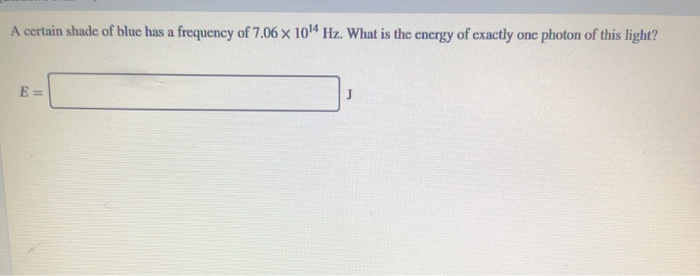A certain shade of blue has a frequency of 7.06 x 104 Hz. What is the energy of exactly one photon of this light? X E= J For a hydrogen-like atom, classify the clectron transitions according to whether they result in the absorption or emission of light. Absorption Emission Answer Bank n-2 to n-1 n-3 to n-2 3 to n n-I to n 3 Ignoring sign, which transition is associated with the greatest energy change? O n 2 ton 1...

• ### Which of the following electron transitions will result in emission of light with the largest wavelength...Which of the following electron transitions will result in emission of light with the largest wavelength Choose One On-1 to n-6 On-Ston-6 On-6 ton-1 On-2 to - 1 On-4to n-3 If the n-5 to the n-2 electron transition in a hydrogen atom occurs at 434 nm, violet is in the range of visible light Sort the following transitions into their likely category. Only one transition should be sorted into each category. Items (4 items) Drag and drop into the appropriate...

• ### transition Of the following transitions in the Bohr hydrogen atom, the_ results in the emission of...transition Of the following transitions in the Bohr hydrogen atom, the_ results in the emission of the lowest-energy photon. When the electron in a hydrogen atom moves from n = 6 ton = 2, light with a wavelength of nm is emitted.

• ### classify statements about this absorption and emission process as true or false An electron in a...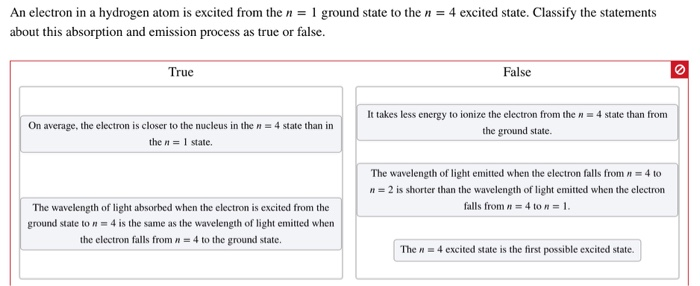classify statements about this absorption and emission process as true or false An electron in a hydrogen atom is excited from the n = 1 ground state to the n = 4 excited state. Classify the statements about this absorption and emission process as true or false. True False On average, the electron is closer to the nucleus in the n = 4 state than in the n = 1 state. It takes less energy to ionize the electron from...

• ### classify statements about absorption and emission process as true or false An electron in a hydrogen...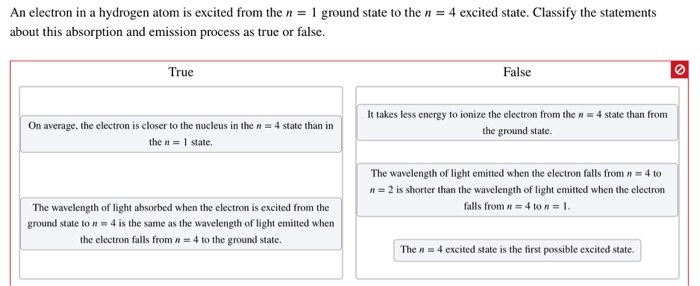classify statements about absorption and emission process as true or false An electron in a hydrogen atom is excited from the n ground state to the n4 excited state. Classify the statements about this absorption and emission process as true or false. True False It takes less energy to ionize the electron from the n4 state than from On average, the electron is closer to the nucleus in the n 4 state than in the ground state. the n 1...

• ### Classify each of the hydrogen atom transitions above. Put letters in alphabetical order (no spaces or...Classify each of the hydrogen atom transitions above. Put letters in alphabetical order (no spaces or commas). If none are correct enter NONE. 1. Absorption 2-Emission 3, Ionization

• ### 1. The figure below illustrates two electron transitions for a hydrogen atom. The transitions are labeled...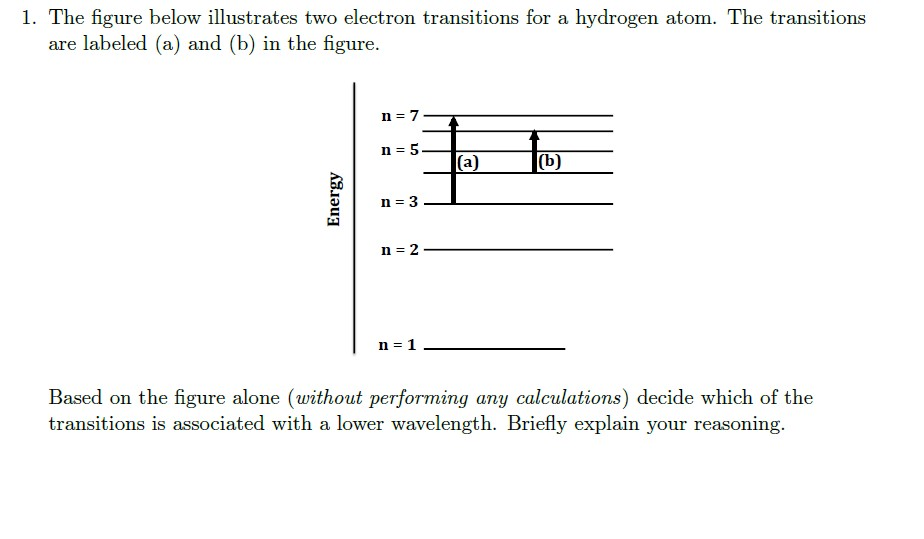1. The figure below illustrates two electron transitions for a hydrogen atom. The transitions are labeled (a) and (b) in the figure. Energy n = 3 - n = 2 1 n = 1 Based on the figure alone (without performing any calculations) decide which of the transitions is associated with a lower wavelength. Briefly explain your reasoning. 2. Use the Balmer-Rydberg equation to calculate the wavelength, la, for transition (a) in the figure above. Calculate the energy, in units...

• ### Compare photons of ultraviolet and infrared radiation. Which has the longer wavelength? Which has the greater...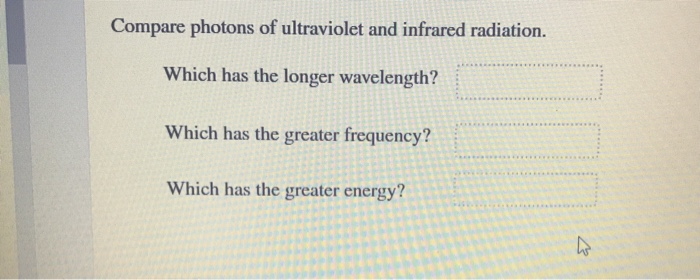Compare photons of ultraviolet and infrared radiation. Which has the longer wavelength? Which has the greater frequency? Which has the greater energy? For a hydrogen-like atom, classify the electron transitions according to whether they result in the absorption or emission of light. Absorption Emission Answer Bank .-2000-1 -- 361-2 -- 16on-3 --3won=5 R 2 - 1 10 - 1 -3

• ### JIIVIILIT NISLI UCCION13 Calculate the energy changes corresponding to the transitions of the hydrogen atom according...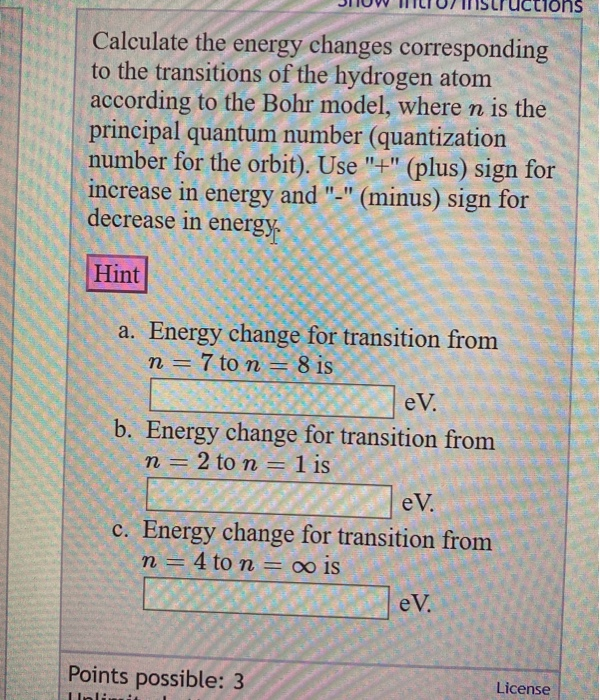JIIVIILIT NISLI UCCION13 Calculate the energy changes corresponding to the transitions of the hydrogen atom according to the Bohr model, where n is the principal quantum number (quantization number for the orbit). Use "+" (plus) sign for increase in energy and "-" (minus) sign for decrease in energy Hint a. Energy change for transition from n = 7 to n = 8 is V. b. Energy change for transition from n = 2 to n = 1 is eV. c....

Free Homework App

Scan Your Homework
to Get Instant Free Answers
Need Online Homework Help?

Get Answers For Free
Most questions answered within 3 hours.